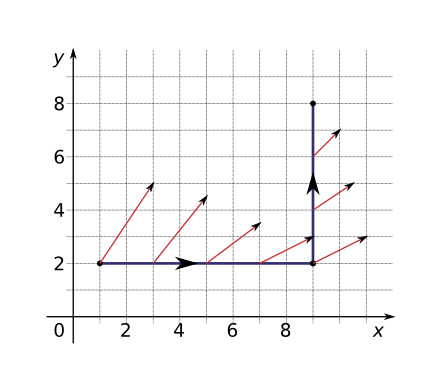Line Integrals

(requires JavaScript)

1. Evaluate the line integral ${\int }_{C}y\phantom{\rule{0.2em}{0ex}}ds$, where $C$ is the curve $x={t}^{2}$, $y=t$, $0\le t\le 2$.
$\frac{17\sqrt{17}-1}{12}$
2. Evaluate the line integral ${\int }_{C}x{y}^{4}\phantom{\rule{0.2em}{0ex}}ds$, where $C$ is the right half of the circle ${x}^{2}+{y}^{2}=16$.
$1638.4$
3. Evaluate the line integral ${\int }_{C}x{y}^{3}\phantom{\rule{0.2em}{0ex}}ds$, where $C$ is the curve $x=4\mathrm{sin}\left(t\right)$, $y=4\mathrm{cos}\left(t\right)$, $z=3t$, $0\le t\le \pi /2$.
$320$
4. Evaluate the line integral ${\int }_{C}x{e}^{yz}\phantom{\rule{0.2em}{0ex}}ds$, where $C$ is the line segment from $\left(0,0,0\right)$ to $\left(1,2,3\right)$.
$\frac{\sqrt{14}\left({e}^{6}-1\right)}{12}$
5. Evaluate the line integral ${\int }_{C}xy\phantom{\rule{0.2em}{0ex}}dx+\left(x-y\right)\phantom{\rule{0.2em}{0ex}}dy$ where $C$ consists of line segments from $\left(0,0\right)$ to $\left(2,0\right)$ and from $\left(2,0\right)$ to $\left(3,2\right)$.
$\frac{17}{3}$
6. Evaluate the line integral ${\int }_{C}\mathrm{sin}\left(x\right)\phantom{\rule{0.2em}{0ex}}dx+\mathrm{cos}\left(y\right)\phantom{\rule{0.2em}{0ex}}dy$ where $C$ consists of the top half of the unit circle from $\left(1,0\right)$ to $\left(-1,0\right)$ and the line segment from $\left(-1,0\right)$ to $\left(-2,3\right)$.
7. Evaluate the line integral ${\int }_{C}{x}^{2}\phantom{\rule{0.2em}{0ex}}dx+{y}^{2}\phantom{\rule{0.2em}{0ex}}dy+{z}^{2}\phantom{\rule{0.2em}{0ex}}dz$, where $C$ consists of line segments from $\left(0,0,0\right)$ to $\left(1,2,-1\right)$, and from $\left(1,2,-1\right)$ to $\left(3,2,0\right)$.
8. Evaluate the line integral ${\int }_{C}\mathbf{F}•\phantom{\rule{0.2em}{0ex}}d\mathbf{r}$ where $\mathbf{F}\left(x,y\right)={x}^{2}{y}^{3}\mathbf{i}-y\sqrt{x}\mathbf{j}$ and $C$ is given by the vector function $\mathbf{r}\left(t\right)={t}^{2}\mathbf{i}-{t}^{3}\mathbf{j}$, $0\le t\le 1$.
$-\frac{59}{105}$
9. Evaluate the line integral ${\int }_{C}\mathbf{F}•\phantom{\rule{0.2em}{0ex}}d\mathbf{r}$ where $\mathbf{F}\left(x,y,z\right)=⟨z,y,-x⟩$ and $C$ is given by the vector function $\mathbf{r}\left(t\right)=⟨t,\mathrm{sin}\left(t\right),\mathrm{cos}\left(t\right)⟩$, $0\le t\le \pi$.
10. A thin wire is bent into the shape of a semicircle ${x}^{2}+{y}^{2}=4$, $x\ge 0$. If the linear density is a constant $k$, find the mass and the centroid of the wire.
$2\pi k$ and $\left(4/\pi ,0\right)$
11. Find the work done by the force field $\mathbf{F}\left(x,y,z\right)=⟨y+z,x+z,x+y⟩$ on a particle that moves along the line segment from $\left(1,0,0\right)$ to $\left(3,4,2\right)$.
$26$
1. An intelligent robot ($160$ kg) is carrying a canister with oil ($25$ kg) up a helical ramp that encircles a rocket with a radius $20$ m. If the rocket is $90$ m high and the robot makes exactly $3$ complete revolutions, how much work is done by the robot against gravity while climbing to the top?
2. If there is a hole in the canister and $9$ kg of oil leaks out at a steady rate during the ascent, how much work is done?
12. An object moves along the curve $C$ shown in the figure from $\left(1,2\right)$ to $\left(9,8\right)$. The lengths of the vectors in the force field $\mathbf{F}$ are measured in newtons by the scales on the axes. Estimate the work done by $\mathbf{F}$ on the object.$22$ J